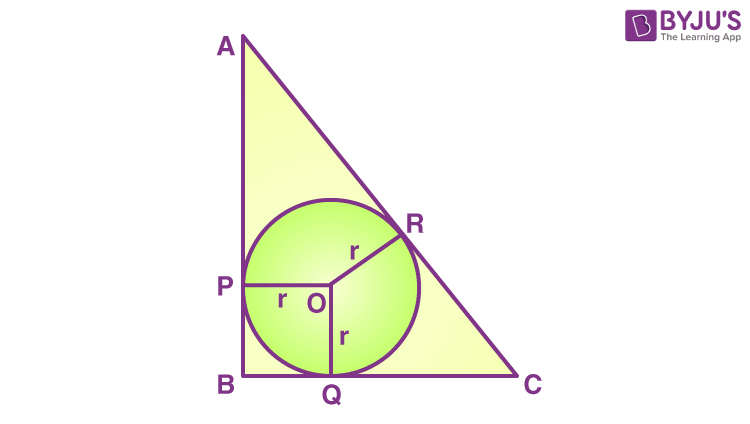# ABC is a right-angled triangle right angled at B such that BC = 6 cm and AB = 8 cm. A circle with center O is inscribed in triangle ABC. The radius of the circle is

(a) 1 cm

(b) 2 cm

(c) 3 cm

(d) 4 cm

Solution:Given BC = 6 cm and AB = 8 cm

By using Pythagoras Theorem, we can find AC.

AC2 = BC2 + AB2

=> AC = √(36+64)

= 10 cm

Let OP = OR = OQ = r

We know that two tangents drawn from an external point to a circle are equal in measure.

BP = BQ

CQ = CR

AP = AR

OP and OQ are radii of the circle.

OP ⊥ AB and OQ ⊥ BC and ∠B = 90° (given)

Hence, BPOQ is a square.

Thus, BP = BQ = r (sides of a square are equal)

So, AR = AP = AB – BP

= 8 – r

CR = CQ

= BC – BQ

= 6 – r

AR + CR = AC

=> 8 – r + 6 – r = 10

=> 14 – 2r = 10

=> 4 = 2r

=> r = 2

Hence option b is the answer.(59)(11)